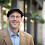## Tuesday, January 28, 2014

### Geometry Problem 970: Equilateral Triangle, Circumcircle, Secant, Metric Relations

Geometry Problem
Level: Mathematics Education, High School, Honors Geometry, College.

Click the figure below to see the complete problem 970.1.Let BD=y.

DE×DA=DB×DC
y(x+y)=12

In ΔABD, using cosine law,
36=x²+y²+xy=x²+y(x+y)=x²+12

x²=24
x=2√6

2.Let BD = p

From similar triangles p(p+x) = 2(2+4) = 12...(1)
From Pythagoras AD^ 2 = 3x^2/4 + (p+x/2)^2 ....(2)

(2) - (1) ; x^2 = AD^2 - 12 = 24

so x = sqrt 24 = 2sqrt6

3.AEBC is a cyclic quad from that 3 similar triangles fall out: ABE, CDE and ABD. Note angles AEC = CEB = DEB = 60 degrees.

Consider just ABE and ABD since they're similar from those we get 4/x = x/6
and x^2 = 24 => x = 2 sqrt(6)

4.Let BD=y
As DE.DA=DB.DC
=> 12=y.(x+y)=> y^2+xy=12
Let F be the foot of perpendicular from A to BC
AF^2+DF^2=DA^2
=>3/4x^2+x^2/4+y^2+xy=36
=>x^2=24